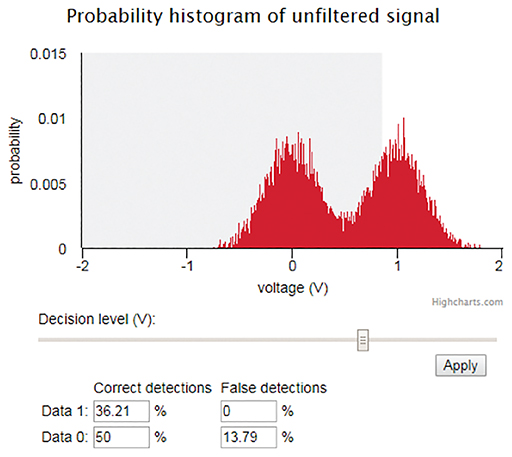Science, Maths & Technology

### Become an OU studentElectronic applications

Start this free course now. Just create an account and sign in. Enrol and complete the course for a free statement of participation or digital badge if available.

# 3.11 Changing the decision level

Again, using the interactive in Section 3.9 you will now look at changing the decision level.

The decision level is represented by the right-hand vertical edge of the grey area on the histogram display. Use the Decision level (V) slider to set the decision level midway between 0 and 1. You will have to judge its position by eye. Click on Apply to implement the decision level and look at the statistics.

## Activity 10

• a.Why are there are false detections?
• b.Why are the detection rates (correct and false) practically the same for 0 and 1?

• a.The effect of noise is to take the signal occasionally over the decision level, so that a 0 is detected as a 1 and vice versa.
• b.The percentages of correct and false detections are virtually the same for 0s and 1s because of the symmetry of the situation. The noise affects the 0s and 1s equally, and the decision level is symmetrically placed between 0 and 1.

The symmetrical arrangement that you have used so far, with the decision level halfway between the two binary symbols, is typical of much practical implementation of binary signal detection, but looking at an asymmetrical arrangement is instructive.

Use the Decision level (V) slider to place the decision level asymmetrically between 0 and 1 (that is, much closer to 1 than to 0, or vice versa), then click on Apply.

## Activity 11

Explain, in general terms, the correct and false detection statistics that have resulted from your asymmetrical placement. You will not be able to give a precise account, but you might be able to explain the relative sizes of the statistics.